# How Artificial Neural Network Work

## How does Artificial Neural Network (ANN) Work?

In this article, I am going to discuss How does Artificial Neural Networks (ANN) Work. Please read our previous article where we discussed Activation Functions in Artificial Neural Networks.

##### How does Artificial Neural Network (ANN) Work?

The development of Artificial Neural Network (ANN) algorithms, which may be applied to model complicated patterns and solve prediction issues, is based on how the brain processes information. Let’s start by comprehending how the brain interprets information.

The neurons in our brain, which number in the billions, process information as electric signals. The dendrites of the neuron take in external information or stimuli, which are then processed in the neuron cell body and transformed into an output before being transmitted along the axon to the following neuron. Depending on the signal’s intensity, the following neuron can decide whether to accept or reject the signal.

Let’s now attempt to comprehend how an ANN functions:
Step 1: Gather the data
Step 2: Processing the input
Step 3: Transmission and processing of the output
Step 4: Collect the results

Now, let’s have a look at how a neural network works –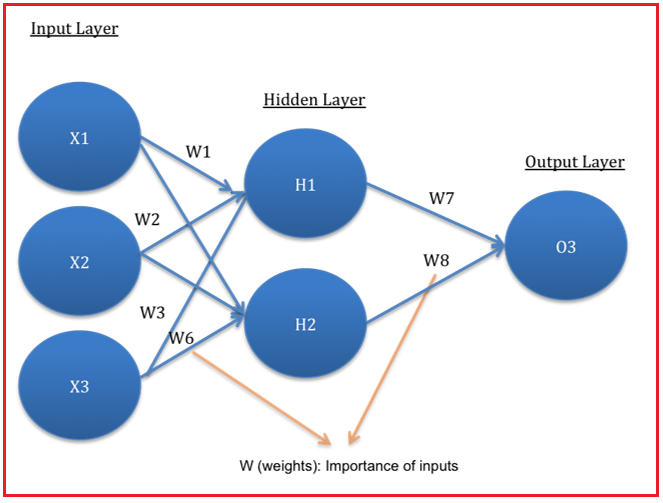1. The network design consists of an input layer, one or more hidden layers, and an output layer. Because there are numerous layers, it is also known as a “Multi-Layer Perceptron.”

2. The hidden layer can be thought of as a “distillation layer” that extracts some of the key input patterns and passes them on to the next layer for detection. By separating out the redundant information from the inputs and identifying only the vital information, it accelerates and optimizes the network.

3. The sigmoid activation function has two noteworthy functions.

• It depicts the non-linear relationship between the inputs.
• It aids in transforming the input into a more beneficial output.

The activation function in the above example is sigmoid. Let’s see what the calculations for this above neural network look like –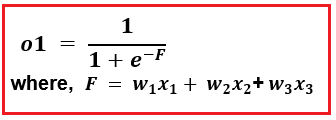4. Similar to this, the hidden layer leads to the output layer’s final prediction: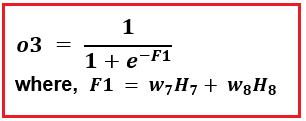The output value (O3) in this case ranges from 0 to 1. A value that is nearer to 1 (for example, 0.75), suggests that there is a greater likelihood of customer default.

5. The weights W indicate the inputs’ relevance.

6. As you can see, the network architecture described above is a “feed-forward network,” as input signals are only flowing in one direction (from inputs to outputs). Additionally, we are able to build “feedback networks with bidirectional signal flow.

7. Predictions from a decent model with high precision are usually fairly accurate. Finding “optimal values of weights” that reduce the prediction error is essential to getting a good model with accurate predictions. This is accomplished using the “Backpropagation algorithm” which transforms ANN into a learning algorithm because the model is enhanced by learning from mistakes.

8. Gradient descent is the most well-known optimization algorithm technique, which employs iteratively varying values of W while evaluating prediction errors. In order to obtain the ideal W, W’s values are modified slightly while the impact on prediction errors is evaluated. Finally, those values of W are selected as optimal where further modifications in W do not result in further reductions in errors.

##### How does Backpropagation work?

The key to creating a trustworthy model is adequate Neural Network training. Most individuals who are learning about deep learning find the word “back-propagation,” which is typically linked with this training, to be extremely ambiguous. Heck, most professionals in the field simply accept the fact that it operates.

The foundation of neural net training is back-propagation. It is the process of adjusting a neural network’s weights based on the error rate (or loss) recorded in the previous epoch (i.e. iteration). Lower error rates are ensured through proper weight adjustment, which broadens the model’s applicability and makes it more dependable.

Neural Networks Function by:

1. Initializing the weights with random numbers, most of which range from 0 to 1
2. To determine the loss or error term, compute the output.
3. After that, change the weights to reduce the loss.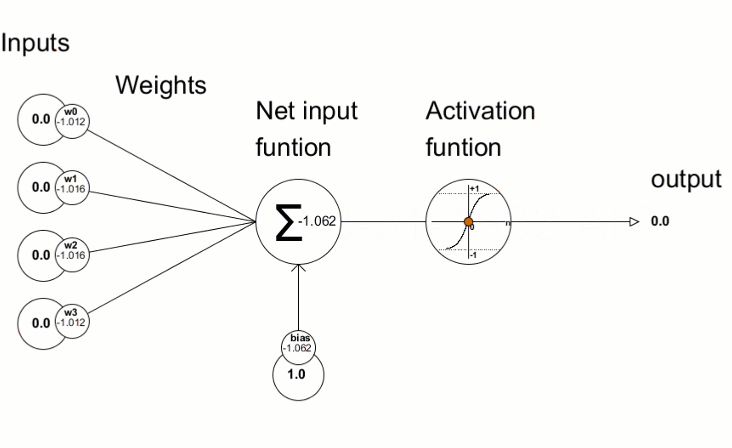Once the minimum loss function has been optimally solved or all predefined epochs have been used, we repeat these three procedures (i.e. the number of iterations). Our objective is to reduce the error, which is obviously reliant on Y, the actual observed data, and on the output, which is additionally dependent on the:

1. input data
2. betas or coefficients of the input variables
3. Activation function, biases, and
4. Optimizers

Now, we have no control over the input variables or the actual Y values, but we do have control over the other variables. The tuning parameters are the activation function and the optimizers, which we can modify based on our needs.

Using the gradient descent process, the other two factors—the biases b and the coefficients or betas of the input variables W are updated.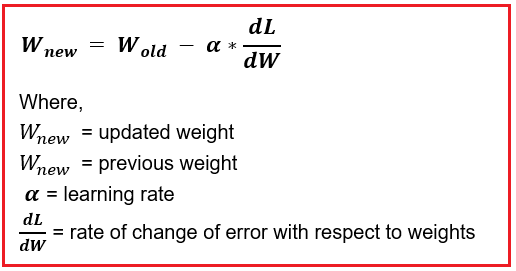We modify these weights or the output betas in the backward propagation. the corresponding input, hidden, and output layers’ weights and biases.

We initialize the weights at random in the first iteration. The hidden layer’s weights that are closest to the output layer are modified in the second iteration. In this instance, the output layer is followed by the hidden layer, and finally the input layer.

##### Illustrate Forward pass, Backward pass

Let’s consider an example of a neural network, to understand how Forward Pass and Backward Pass actually work.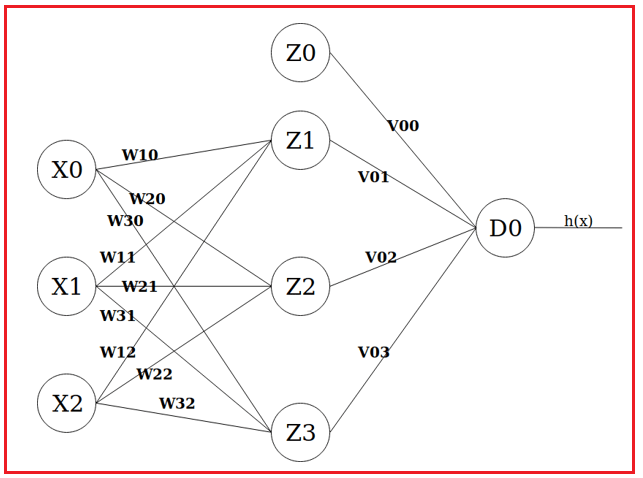The information should now be fed forward from one layer to the next. There are two steps in this process that occur at each node/unit in the network:

1. Using the h(x) function we previously established, calculate the weighted sum of inputs for a specific unit.
2. Using the value, we obtain from step 1 as the input feature for the connected nodes in the following layer, we plug it into the activation function we have (f(a)=a in this case) and obtain the activation value.

So, let’s see what the mathematics for this neural network looks like –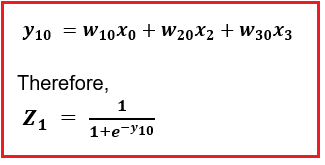The calculations for and give similar values. Now, let’s move to calculations for the output layer.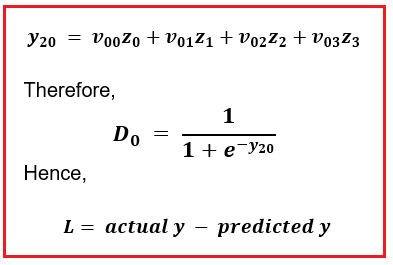Now, the backward propagation begins from right to left. Let’s calculate derivatives for weights of the hidden layer.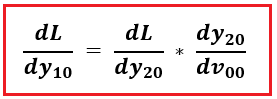Similarly, we can calculate derivatives for the rest of the weights of hidden layers too. And then update weights accordingly. After the hidden layer, we will move towards the hidden layer to get the weights updated. Example –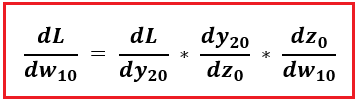Now, once we have all the derivatives, we can update new weights by using different optimizers like gradient descent.

In the next article, I am going to discuss Gradient Descent in Artificial Neural Networks. Here, in this article, I try to explain the How do Artificial Neural Networks (ANN) Work. I hope you enjoy this How do Artificial Neural Network Work article. Please post your feedback, suggestions, and questions about this How do Artificial Neural Networks (ANN) Work article.

### 1 thought on “How Artificial Neural Network Work”

1.Guys,
Please give your valuable feedback. And also, give your suggestions about How does Artificial Neural Networks (ANN) Work concept. If you have any key points related to How does Artificial Neural Networks (ANN) Work, you can also share the same.